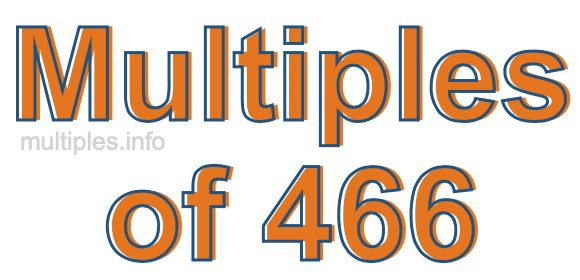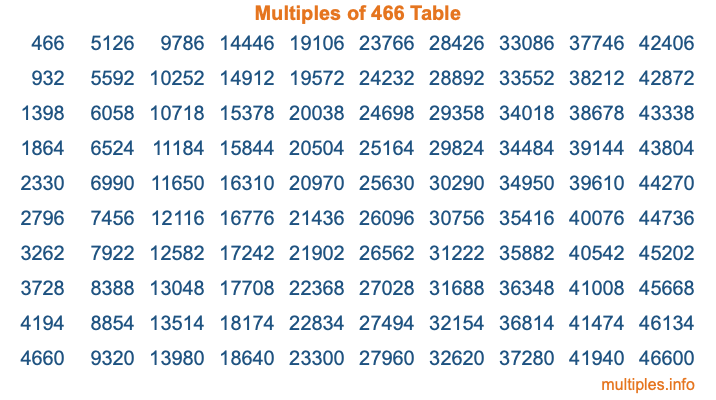Multiples of 466Welcome to the Multiples of 466 page. Here we will first teach you everything you will ever need to know about the multiples of 466, and then give you a study guide summary of everything we taught you to make sure you remember it all. Use this page to look up facts and learn information about the multiples of 466. This page will make you a multiples of four hundred sixty-six expert!

Definition of Multiples of 466
Multiples of 466 are all the numbers that when divided by 466 equal an integer. Each of the multiples of 466 are called a multiple. A multiple of 466 is created by multiplying 466 by an integer.

Therefore, to create a list of multiples of 466, you start with 1 multiplied by 466, then 2 multiplied by 466, then 3 multiplied by 466, and so on for as long as you want. Thus, the list of the first five multiples of 466 is 466, 932, 1398, 1864, and 2330. To see a larger list of multiples of 466, see the printable image of Multiples of 466 further down on this page. We also have a category where you can choose any nth multiple of 466.

Multiples of 466 Checker
The Multiples of 466 Checker below checks to see if any number of your choice is a multiple of 466. In other words, it checks to see if there is any number (integer) that when multiplied by 466 will equal your number. To do that, we divide your number by 466. If the the quotient is an integer, then your number is a multiple of 466.

Is  a multiple of 466?

Least Common Multiple of 466 and ...
A Least Common Multiple (LCM) is the lowest multiple that two or more numbers have in common. This is also called the smallest common multiple or lowest common multiple and is useful to know when you are adding our subtracting fractions. Enter one or more numbers below (466 is already entered) to find the LCM.

Check out our LCM Calculator if you need more details about the Least Common Multiple or if you need the LCM for different numbers for adding and subtraction fractions.

nth Multiple of 466
As we stated above, 466 is the first multiple of 466, 932 is the second multiple of 466, 1398 is the third multiple of 466, and so on. Enter a number below to find the nth multiple of 466.

th multiple of 466

Multiples of 466 vs Factors of 466
466 is a multiple of 466 and a factor of 466, but that is where the similarities end. All postive multiples of 466 are 466 or greater than 466. All positive factors of 466 are 466 or less than 466.

Below is the beginning list of multiples of 466 and the factors of 466 so you can compare:

Multiples of 466: 466, 932, 1398, 1864, 2330, etc.

Factors of 466: 1, 2, 233, 466

As you can see, the multiples of 466 are all the numbers that you can divide by 466 to get a whole number. The factors of 466, on the other hand, are all the whole numbers that you can multiply by another whole number to get 466.

It's also interesting to note that if a number (x) is a factor of 466, then 466 will also be a multiple of that number (x).

Multiples of 466 vs Divisors of 466
The divisors of 466 are all the integers that 466 can be divided by evenly. Below is a list of the divisors of 466.

Divisors of 466: 1, 2, 233, 466

The interesting thing to note here is that if you take any multiple of 466 and divide it by a divisor of 466, you will see that the quotient is an integer.

Multiples of 466 Table
Below is an image of the first 100 multiples of 466 in a table. The table is in chronological order, column by column. The first column has the first ten multiples of 466, the second column has the next ten multiples of 466, and so on.The Multiples of 466 Table is also referred to as the 466 Times Table or Times Table of 466. You are welcome to print out our table for your studies.

Negative Multiples of 466
Although not often discussed or needed in math, it is worth mentioning that you can make a list of negative multiples of 466 by multiplying 466 by -1, then by -2, then by -3, and so on, to get the following list of negative multiples of 466:

-466, -932, -1398, -1864, -2330, etc.

Multiples of 466 Summary
Below is a summary of important Multiples of 466 facts that we have discussed on this page. To retain the knowledge on this page, we recommend that you read through the summary and explain to yourself or a study partner why they hold true.

There are an infinite number of multiples of 466.

A multiple of 466 divided by 466 will equal a whole number.

466 divided by a factor of 466 equals a divisor of 466.

The nth multiple of 466 is n times 466.

The largest factor of 466 is equal to the first positive multiple of 466.

466 is a multiple of every factor of 466.

466 is a multiple of 466.

A multiple of 466 divided by a divisor of 466 equals an integer.

466 divided by a divisor of 466 equals a factor of 466.

Any integer times 466 will equal a multiple of 466.

Multiples of a Number
Here you can get the multiples of another number, all with the same attention to detail as we did for multiples of 466 on this page.

Multiples of
Multiples of 467
Did you find our page about multiples of four hundred sixty-six educational? Do you want more knowledge? Check out the multiples of the next number on our list!